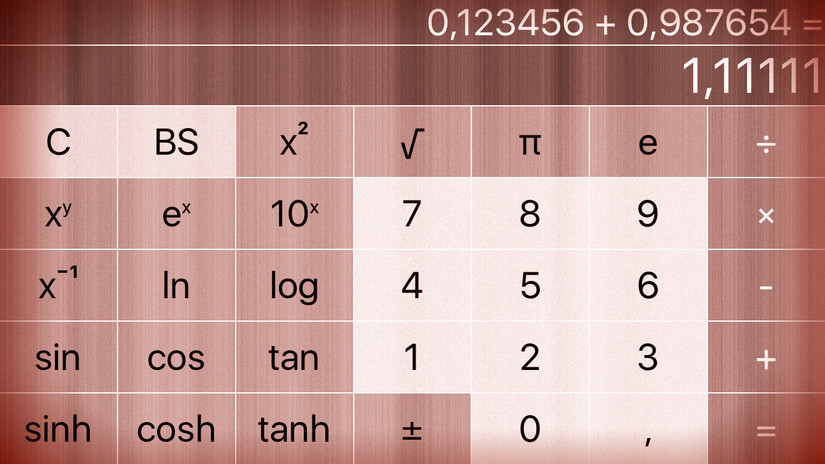# cs193p – Assignment #1 Extra Task #3Please note, this blog entry is from a previous course. You might want to check out the current one.

Figure out from the documentation how to use the NSNumberFormatter class to format your display so that it only shows 6 digits after the decimal point (instead of showing all digits that can be represented in a Double). This will eliminate the need for Autoshrink in your display. While you’re at it, make it so that numbers that are integers don’t have an unnecessary “.0” attached to them (e.g. show “4” rather than “4.0” as the result of the square root of sixteen). You can do all this for your description in the CalculatorBrain as well.

NSNumberFormatter uses country-specific number formats. Meaning if your country uses a comma instead of a dot as decimal separator – and your device is set accordingy – the number format will use that specific format. Up to now we only used a dot as decimal separator. We could continue to do that by forcing the number format e.g. by telling it we are always using the US format, or we just deal with it.

Let’s teal with it, starting by changing the the title of the comma button accordingly. First change the tag of it to “3”, than update its title:

```private let decimalSeparator = NSNumberFormatter().decimalSeparator!

private func adjustButtonLayout(view: UIView, portrait: Bool) {
...
if button.tag == 3 {
button.setTitle(decimalSeparator, forState: .Normal)
}
...
}
```

Adjust the places where you (actually I) have hardcoded the “.”:

```@IBAction private func touchDigit(sender: UIButton) {
...
if digit == decimalSeparator {
...
if !userIsInTheMiddleOfTyping {
digit = "0" + decimalSeparator
}
...
}
```

… and because hardcoding is a bad idea anyway, create a structure of constants holding the number of decimal digits we want to use:

```private struct Constants {
static let DecimalDigits = 6
}
```

To get the display value just use the standard number formatter. To set the display value adjust the formatter to use the maximum number of decimal digits:

```private var displayValue: Double? {
get {
if let text = display.text, value =
NSNumberFormatter().numberFromString(text)?.doubleValue {
...
}
set {
if let value = newValue {
let formatter = NSNumberFormatter()
formatter.numberStyle = .DecimalStyle
formatter.maximumFractionDigits = Constants.DecimalDigits
display.text = formatter.stringFromNumber(value)
...
}
}
```

Unfortunately, the calculator model also needs to be adjusted to use the correct decimal separator and number of digits for the description text – which does not coincide with the MVC model. The decimal separator is derived from the standard number formatter. For the decimal digits we us public property which should be set when the model is generated:

```var decimalDigits: Int

init(decimalDigits:Int) {
self.decimalDigits = decimalDigits
}
```

… and change the description for the operand to use the formatter:

```func setOperand(operand: Double) {
...
let formatter = NSNumberFormatter()
formatter.numberStyle = .DecimalStyle
formatter.maximumFractionDigits = decimalDigits
descriptionAccumulator = formatter.stringFromNumber(operand)!
}
```

Don’t forget the new init method in the view controller:

```brain = CalculatorBrain(decimalDigits: Constants.DecimalDigits)
```

The complete code for the extra task #3 is available on GitHub.

1. Derek says:

Hello